# Depreciation Methods

The four main types

## What Are the Main Types of Depreciation Methods?

There are several types of depreciation expense and different formulas for determining the book value of an asset. The most common depreciation methods include:

1. Straight-line
2. Double declining balance
3. Units of production
4. Sum of years digits

Depreciation expense is used in accounting to allocate the cost of a tangible asset over its useful life. In other words, it is the reduction in the value of an asset that occurs over time due to usage, wear and tear, or obsolescence.  The four main depreciation methods mentioned above are explained in detail below.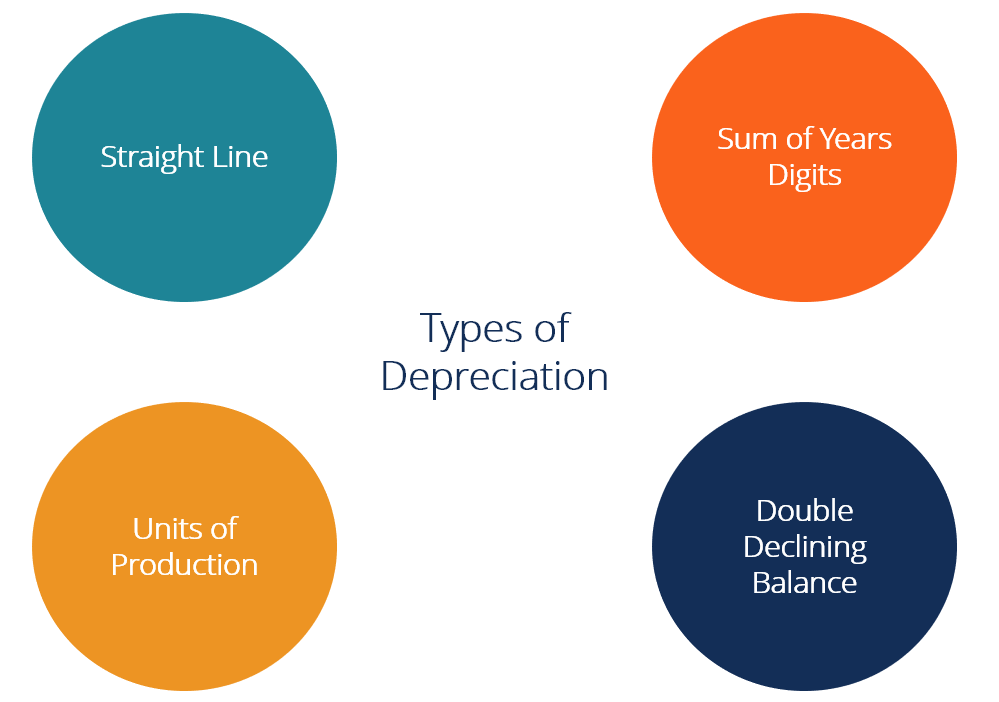### #1 Straight-Line Depreciation Method

Straight-line depreciation is a very common, and the simplest, method of calculating depreciation expense. In straight-line depreciation, the expense amount is the same every year over the useful life of the asset.

Depreciation Formula for the Straight Line Method:

Depreciation Expense = (Cost – Salvage value) / Useful life

##### Example

Consider a piece of equipment that costs \$25,000 with an estimated useful life of 8 years and a \$0 salvage value. The depreciation expense per year for this equipment would be as follows: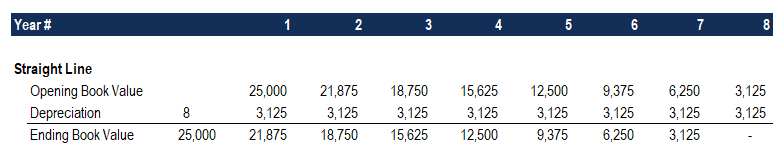Depreciation Expense = (\$25,000 – \$0) / 8 = \$3,125 per year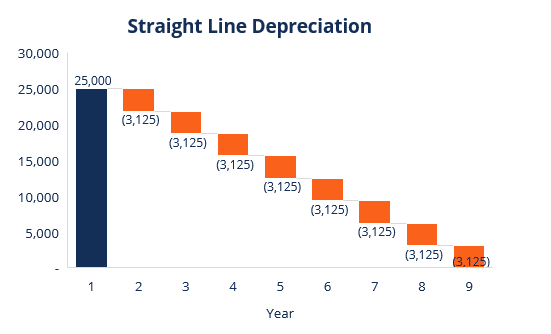### #2 Double Declining Balance Depreciation Method

Compared to other depreciation methods, double-declining-balance depreciation results in a larger amount expensed in the earlier years as opposed to the later years of an asset’s useful life. The method reflects the fact that assets are typically more productive in their early years than in their later years – also, the practical fact that any asset (think of buying a car) loses more of its value in the first few years of its use. With the double-declining-balance method, the depreciation factor is 2x that of the straight-line expense method.

Depreciation formula for the double-declining balance method:

Periodic Depreciation Expense = Beginning book value x Rate of depreciation

##### Example

Consider a piece of property, plant, and equipment (PP&E) that costs \$250,000, with an estimated useful life of 8 years and a \$2,500 salvage value. To calculate the double-declining balance depreciation, set up a schedule: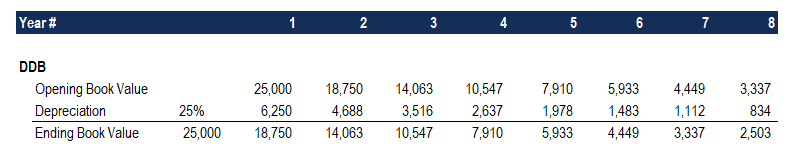The information on the schedule is explained below:

1. The beginning book value of the asset is filled in at the beginning of year 1 and the salvage value is filled in at the end of year 8.
2. The rate of depreciation (Rate) is calculated as follows:

Expense = (100% / Useful life of asset) x 2

Expense = (100% / 8) x 2 = 25%

Note: Since this is a double-declining method, we multiply the rate of depreciation by 2.

3. Multiply the rate of depreciation by the beginning book value to determine the expense for that year. For example, \$25,000 x 25% = \$6,250 depreciation expense.

4. Subtract the expense from the beginning book value to arrive at the ending book value. For example, \$25,000 – \$6,250 = \$18,750 ending book value at the end of the first year.

5. The ending book value for that year is the beginning book value for the following year. For example, the year 1 ending book value of \$18,750 would be the year 2 beginning book value. Repeat this until the last year of useful life.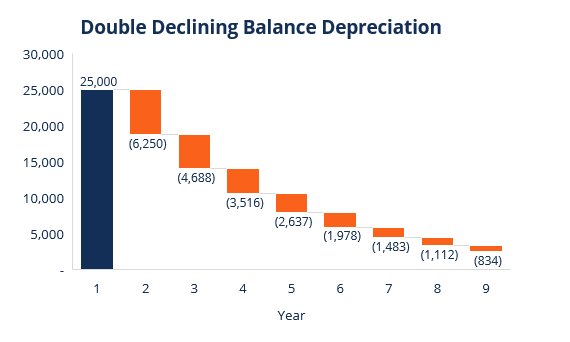### #3 Units of Production Depreciation Method

The units-of-production depreciation method depreciates assets based on the total number of hours used or the total number of units to be produced by using the asset, over its useful life.

The formula for the units-of-production method:

Depreciation Expense = (Number of units produced / Life in number of units) x (Cost – Salvage value)

##### Example

Consider a machine that costs \$25,000, with an estimated total unit production of 100 million and a \$0 salvage value. During the first quarter of activity, the machine produced 4 million units.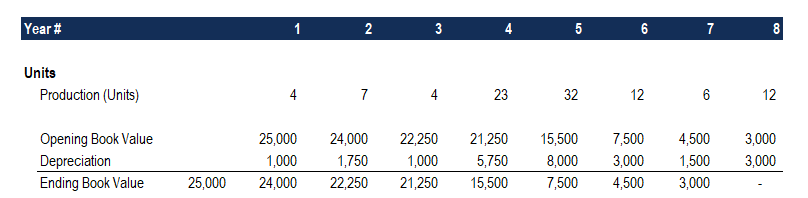To calculate the depreciation expense using the formula above:

Depreciation Expense = (4 million / 100 million) x (\$25,000 – \$0) = \$1,000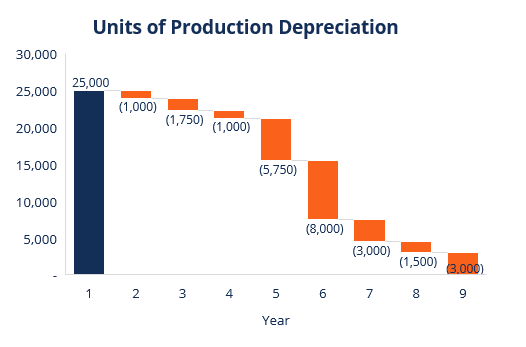### #4 Sum-of-the-Years-Digits Depreciation Method

The sum-of-the-years-digits method is one of the accelerated depreciation methods. A higher expense is incurred in the early years and a lower expense in the latter years of the asset’s useful life.

In the sum-of-the-years digits depreciation method, the remaining life of an asset is divided by the sum of the years and then multiplied by the depreciating base to determine the depreciation expense.

The depreciation formula for the sum-of-the-years-digits method:

Depreciation Expense = (Remaining life / Sum of the years digits) x (Cost – Salvage value)

Consider the following example to more easily understand the concept of the sum-of-the-years-digits depreciation method.

##### Example

Consider a piece of equipment that costs \$25,000 and has an estimated useful life of 8 years and a \$0 salvage value. To calculate the sum-of-the-years-digits depreciation, set up a schedule: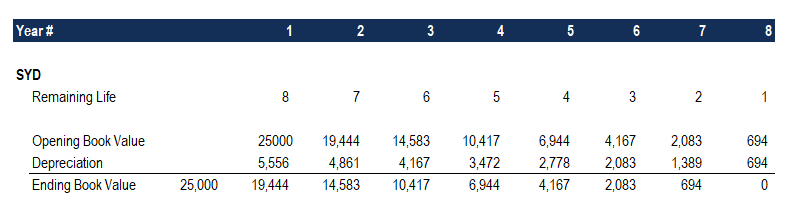The information in the schedule is explained below:

1. The depreciation base is constant throughout the years and is calculated as follows:

Depreciation Base = Cost – Salvage value

Depreciation Base = \$25,000 – \$0 = \$25,000

2. The remaining life is simply the remaining life of the asset. For example, at the beginning of the year, the asset has a remaining life of 8 years. The following year, the asset has a remaining life of 7 years, etc.

3. RL / SYD is “remaining life divided by sum of the years.” In this example, the asset has a useful life of 8 years. Therefore, the sum of the years would be 1 + 2 + 3 + 4 + 5 + 6 + 7 + 8 = 36 years. The remaining life in the beginning of year 1 is 8. Therefore, the RM / SYD = 8 / 36 = 0.2222.

4. The RL / SYD number is multiplied by the depreciating base to determine the expense for that year.

5. The same is done for the following years. In the beginning of year 2, RL / SYD would be 7 / 36 = 0.1944. 0.1944 x \$25,000 = \$4,861 expense for year 2.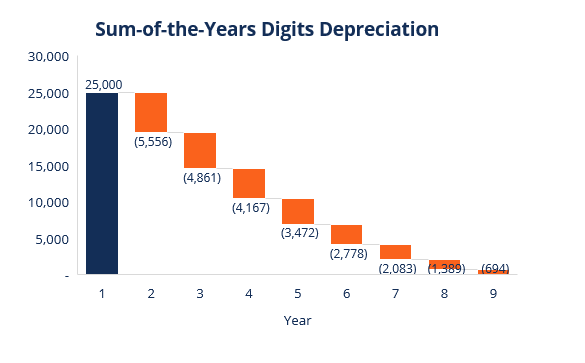### Summary of Depreciation Methods

Below is the summary of all four depreciation methods from the examples above.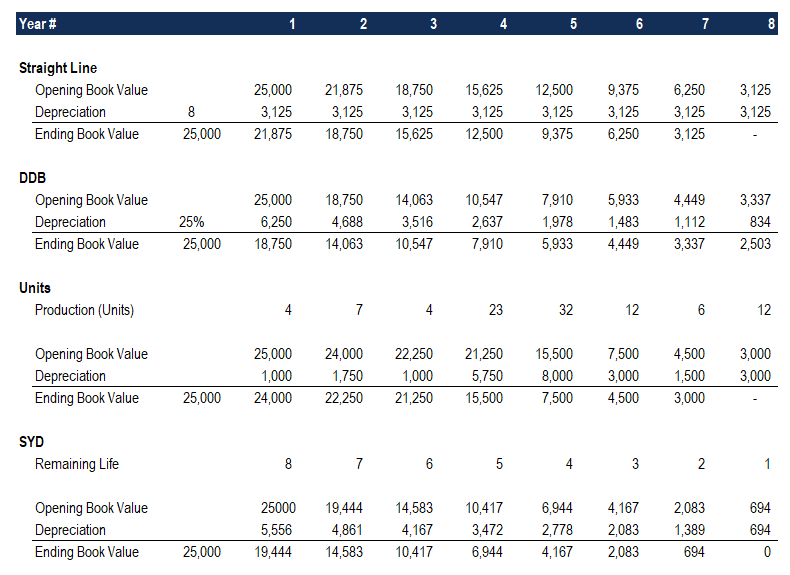Here is a graph showing the book value of an asset over time with each different method.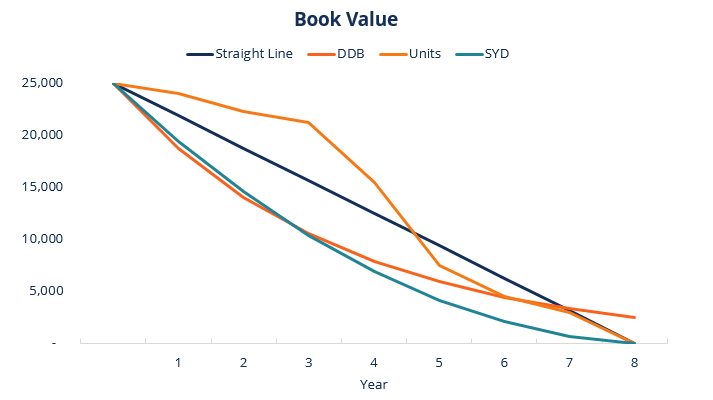Here is a summary of the depreciation expense over time for each of the 4 types of expense.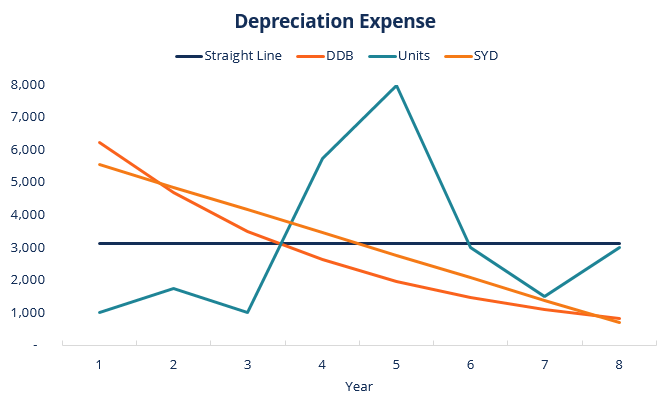### Video Explanation of Depreciation Methods

Below is a short video tutorial that goes through the four types of depreciation outlined in this guide. While the straight-line method is the most common, there are also many cases where accelerated methods are preferable, or where the method should be tied to usage, such as units of production.

Video: CFI’s Financial Analysis Courses.

### More Resources

Thank you for reading this CFI guide to the 4 main types of depreciation.  CFI is a global provider of financial modeling courses and financial analyst certification. To help you become a world-class financial analyst, these additional CFI resources will be helpful:

• Depreciation Schedule
• Depreciation Expense
• Projecting Balance Sheet Items
• Property, Plant & Equipment (PP&E)